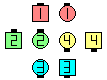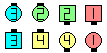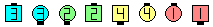Definitions of Square Dance Calls and Concepts

Index -->  Plus  |  A1  |  A2  |  C1  |  C2  |  C3A  |  C3B  |  C4  |  NOL  |
Definitions (Text Only) -->  Plus  |  A1  |  A2  |  C1  |  C2  |  C3A  |  C3B  |  C4  |  NOL  |
 Find call:

\$B0lHL2=\$5\$l\$?(B 1/4 Line \$B\$^\$?\$OB>\$NE,@Z\$J(B formation \$B\$+\$i(B.

Outsides \$B\$O(B Rally \$B\$r\$7(B, Centers \$B\$O(B Step And Cross Fold (Interlocked Little) \$B\$r\$7\$F(B Peel & Trail \$B\$r\$7\$^\$9(B.

\$B0lHL2=\$5\$l\$?(B 1/4 Line \$B\$+\$i\$O(B Tidal Line \$B\$G=*\$o\$j\$^\$9(B. Centers \$B\$K\$H\$C\$F\$O(B 2 \$B%Q!<%H\$N%3!<%k\$G\$9(B.Interlocked Rally\$B\$NA0(B Centers Step And Cross Fold as Ends 1/4 Right & Counter Rotate 1/4(after Interlocked Little)\$B\$N8e(B Centers Peel & Trail as Ends Inward (Right) Roll To A Wave\$B\$N8e(B (\$B=*\$o\$j(B)

\$BCm
• Interlocked Scoot & Rally [C3B] \$B\$G\$O(B, Outsides \$B\$O;X<(\$,\$J\$1\$l\$P(B, \$B;H\$C\$?
• \$BNr;KE*\$K(B, Twin Diamonds \$B\$+\$i\$N(B Interlocked Rally \$B\$G\$O(B, outsides \$B\$K\$O(B 'go As You Are' (\$B\$9\$J\$o\$A(B, 1/4 Right \$B\$r\$;\$:\$K(B) \$B\$,4|BT\$5\$l\$F\$\$\$k\$H\$\$\$&\$3\$H\$,\$"\$j\$^\$7\$?(B. \$B\$3\$N;H\$\$J}\$O(B, \$B:#\$O\$R\$s\$7\$e\$/\$rGc\$&\$b\$N\$G\$9(B. \$B;dC#\$N0U8+\$G\$O(B, \$B%3!<%i!<\$O(B 'Outsides go As You Are' \$B\$d(B 'Centers Start' \$B\$N\$h\$&\$K(B, \$B\$O\$C\$-\$j\$H8@\$&\$Y\$-\$G\$9(B. \$B\$=\$&\$9\$l\$P(B outsides \$B\$O(B 1/4 Right \$B\$G;O\$a\$J\$\$\$G\$7\$g\$&(B.
• Interlocked Rally But anything [C3B]: Interlocked Rally But anything [C3B]: Centers \$B\$O(B, Peel & Trail \$B\$r(B anything call \$B\$G(B Replace \$B\$7\$^\$9(B.

\$BRally [C3A].Choreography for Interlocked RallyComments? Questions? Suggestions?

https://www.ceder.net/def/interlockedrally.php?level=C3B&language=japan
08-February-2023 23:00:54